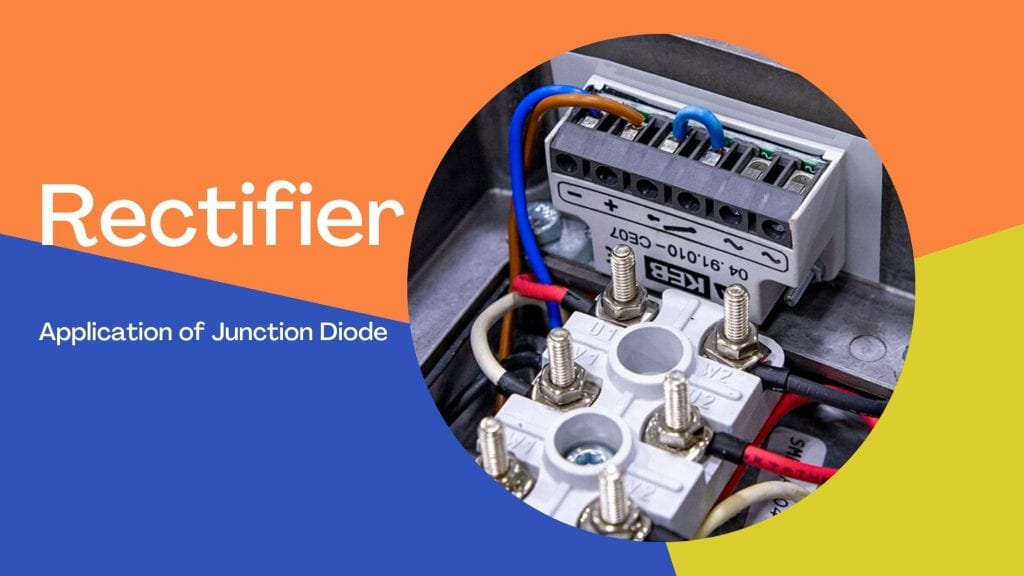Physics

# Rectifier- Application of Junction Diode as Rectifier

Rectifier is a device made of pn junction diode used to convert alternating current to direct current when connected in forward biased condition.

3 minutes long
Posted by Mahak Jain, 7/7/2021Hesap Oluştur

Got stuck on homework? Get your step-by-step solutions from real tutors in minutes! 24/7. Unlimited.

We know that a junction diode allows current to pass only in forward biased condition. So, if we apply alternating voltage across a diode the current flows only in that part of the cycle when the diode is forward biased. This property is used to rectify alternating voltages and the circuit used for this purpose is called a rectifier.

## Half Wave Rectifier

If an alternating voltage is applied across a diode in series with a load, a pulsating voltage will appear across the load. This is only during the half cycle of the ac input during which the diode is forward biased. Such rectifier circuit is a half wave rectifier. The secondary of a transformer supplies the desired ac voltage across terminals A and B. When the voltage at A is positive, the diode is forward biased and it conducts. When A is negative, the diode is reverse-biased and it does not conduct. The reverse saturation current of a diode is negligible and can be considered equal to zero for practical purposes.

Therefore, in the positive half-cycle of ac there is a current through the load resistor RL and we get an output voltage. Whereas, there is no current in the negative half cycle. In the next positive half cycle, again we get the output voltage. Thus, the output voltage, though still varying, is restricted to only one direction and is said to be rectified. Since the rectified output of this circuit is only for half of the input ac wave it is called as half-wave rectifier.

## Full Wave Rectifier

The circuit using two diodes, shown in Fig. 14.19(a), gives output rectified voltage corresponding to both the positive as well as negative half of the ac cycle. Hence, it is known as full-wave rectifier. Here the p-side of the two diodes are connected to the ends of the secondary of the transformer. The n-side of the diodes are connected together and the output is taken between this common point of diodes. The midpoint of the secondary of the transformer. So for a full-wave rectifier the secondary of the transformer is provided with a centre tapping and so it is called centre-tap transformer. As can be seen from Fig.14.19(c) the voltage rectified by each diode is only half the total secondary voltage. Each diode rectifies only for half the cycle, but the two do so for alternate cycles.

So, diode D1 gets forward biased and conducts (while D2 being reverse biased is not conducting). Hence, during this positive half cycle we get an output current (and a output voltage across the load resistor RL) as shown in Fig.14.19(c). In the course of the ac cycle when the voltage at A becomes negative with respect to centre tap, the voltage at B would be positive. In this part of the cycle diode D1 would not conduct but diode D2 would, giving an output current and output voltage (across RL) during the negative half cycle of the input ac. Thus, we get output voltage during both the positive as well as the negative half of the cycle. Obviously, this is a more efficient circuit for getting rectified voltage or current than the half wave rectifier.